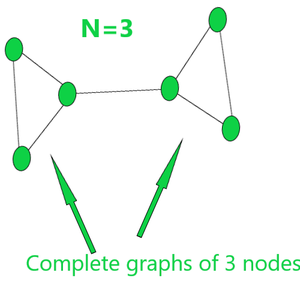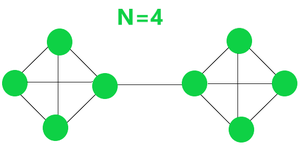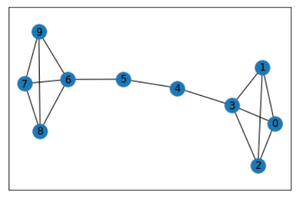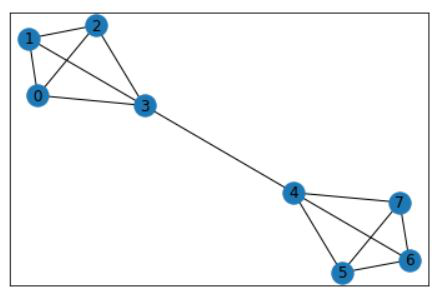GeeksforGeeks App
Open AppBrowser
Continue

# Barbell Graph Using Python networkx

Prerequisite: networkx

There are many kinds of definitions of the barbell graphs. The most commonly used one is an n-barbell graph which is a simple graph obtained by connecting two copies of a complete graph with n nodes. In this article, we are going to see how to use a barbell graph using python.

Examples of n-barbell graph:

Example 1:

If N=3 Nodes then the graph will be shown as figures:Example 2:

If N=4 Nodes then the graph will be shown as figures:#### Analysis:

1.Total number of nodes(In n-barbell graph):

`The Total number of Nodes = 2*N`

2.Total number of edges(In n-barbell graph):

```Total number of edges = 2*number of edgesin complete graph + 1
=2*(n*(n-1)/2)+1 = n*(n-1) + 1```

Properties:

1. The barbell graph contains cycles in it.
2. The barbell graph is connected every two nodes have a path between them.
3. It has a bridge between 2 complete graphs.
4. Bridge may and may not have nodes in it.

Barbell Graphs using Python:

It is realized in python using the barbell_graph(n, m) function of the networkx library and matplotlib library.

• networkx library  Library in python used for realizing and analyzing different kinds of graphs(data structure) in python. For installation use this command:
`pip install networkx`
• matplotlib library: Library in python used for realizing and analyzing different kinds of functions in python. For installation use this command:
`pip install matplotlib`

barbell_graph(n, m): It returns a Barbell Graph with two complete graphs of n nodes which are connected via m node bridge in between.

Approach:

• Import networkx and matplotlib libraries.
• Create a networkx graph object G using nx.barbell_graph(n, m) function as mentioned above.
• Use nx.draw_networkx(G) function to print the graph.

Example 1:

## Python

 `# import module``import` `networkx as nx  ``import` `matplotlib.pyplot as plt `` ` `# graph created``res ``=` `nx.barbell_graph(``4``, ``2``) ``nx.draw_networkx(res)`Barbell Graph in Python

Explanation:

As we passed (4,2) as parameter’s to nx.barbell_graph() function is assigned a graph with 4 node clusters joined by a bridge of 2 nodes. And finally, we got the output as a view of graph G using the draw_networkx(G) function.

Example 2:

## Python3

 `import` `networkx as nx  ``import` `matplotlib.pyplot as plt`` ` `res ``=` `nx.barbell_graph(``4``, ``0``) ``nx.draw_networkx(res)`

Output:My Personal Notes arrow_drop_up